## Crooked coin: Solution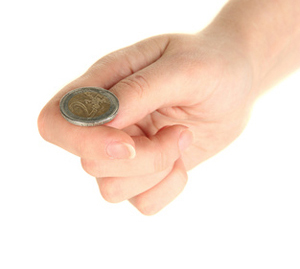Two people have asked you out for a date and you've decided to decide who to go with by flipping a coin. The problem is that the only coin you have is biased: you know that heads comes up with a probability of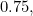rather than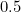as is the case with a fair coin. However, you do really want each of the two people to have a fair chance of being picked. Using a combination of two coin flips instead of one, can you find a way of making the random decision fair?

(Hint: the chance doesn't have to be 50:50.)

### Solution

Make your decision as follows: if in two flips the coin comes up heads first and then tails, go with person A. If it comes up tails first and then heads, go with person B. If it comes up both heads or both tails, ignore the flips and try again.

The probability of going with person A is now the probability that heads comes up times the probability that tails comes up, so that’s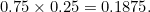The probability of going with person B is the probability that tails comes up times the probability that heads comes up, which is of course the same as the probability of going with person A: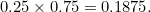You might have to flip for a while, since you have to ignore the heads-heads and tails-tails outcomes, but at least you can be sure that A and B have the same chance of being picked.

The same works for any pair of probabilities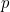for heads and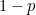for tails. In each case the chance of each person being picked is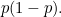Back to puzzle

• Want facts and want them fast? Our Maths in a minute series explores key mathematical concepts in just a few words.